# Changing Themes (Look and Feel) in ggplot2 in R

The themes layer is used to style all the non-data ink of the plot, i.e., all the visual elements that are not part of data.

When we create a chart using ggplot2, it automatically uses a default theme. For theming we have a few choices:

1. Use the default theme (What we have seen so far). The default theme is called theme_grey().
2. Use a built-in theme. There are two built-in themes 1) theme_grey() which is default and 2) theme_bw(), a theme with a white background.
3. Modify/override specific elements of the default theme. This is done by using the theme() function. It overrides the graphical parameters of the default theme.

### Use the Default Theme

The following chart is created using the default theme:

g <- ggplot(df,aes(x=Duration.of.Credit..in.months.,y=Credit.amount,color=Loan.Quality))
g+geom_point()+geom_smooth()+
facet_wrap(~Type.of.housing)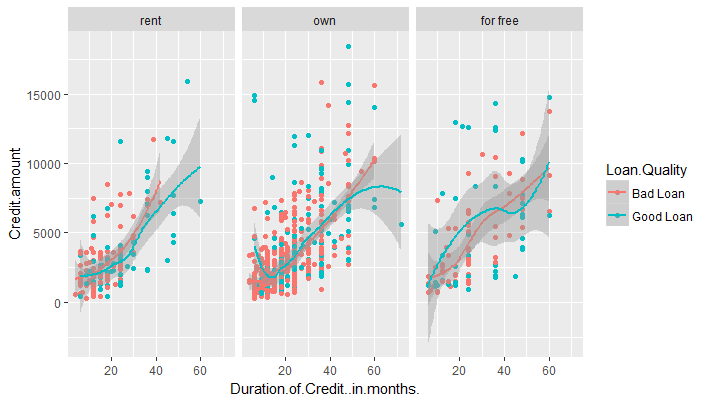Here is the definition of theme_gray theme, straight from ggplot2's source code:

theme_gray <- function(base_size = 12) {
structure(list(
axis.line =         theme_blank(),
axis.text.x =       theme_text(size = base_size * 0.8 , lineheight = 0.9, colour = "grey50", vjust = 1),
axis.text.y =       theme_text(size = base_size * 0.8, lineheight = 0.9, colour = "grey50", hjust = 1),
axis.ticks =        theme_segment(colour = "grey50"),
axis.title.x =      theme_text(size = base_size, vjust = 0.5),
axis.title.y =      theme_text(size = base_size, angle = 90, vjust = 0.5),
axis.ticks.length = unit(0.15, "cm"),
axis.ticks.margin = unit(0.1, "cm"),
legend.background = theme_rect(colour="white"),
legend.key =        theme_rect(fill = "grey95", colour = "white"),
legend.key.size =   unit(1.2, "lines"),
legend.text =       theme_text(size = base_size * 0.8),
legend.title =      theme_text(size = base_size * 0.8, face = "bold", hjust = 0),
legend.position =   "right",
panel.background =  theme_rect(fill = "grey90", colour = NA),
panel.border =      theme_blank(),
panel.grid.major =  theme_line(colour = "white"),
panel.grid.minor =  theme_line(colour = "grey95", size = 0.25),
panel.margin =      unit(0.25, "lines"),
strip.background =  theme_rect(fill = "grey80", colour = NA),
strip.text.x =      theme_text(size = base_size * 0.8),
strip.text.y =      theme_text(size = base_size * 0.8, angle = -90),
plot.background =   theme_rect(colour = NA, fill = "white"),
plot.title =        theme_text(size = base_size * 1.2),
plot.margin =       unit(c(1, 1, 0.5, 0.5), "lines")
), class = "options")}


### Use a built-in Theme

We can use another built-in theme by adding the theme name to the plot function using a + sign.

The theme_bw() function produces a mostly (but not entirely) black-and-white theme.

g <- ggplot(df,aes(x=Duration.of.Credit..in.months.,y=Credit.amount,color=Loan.Quality))
g+geom_point()+geom_smooth()+facet_wrap(~Type.of.housing)+theme_bw()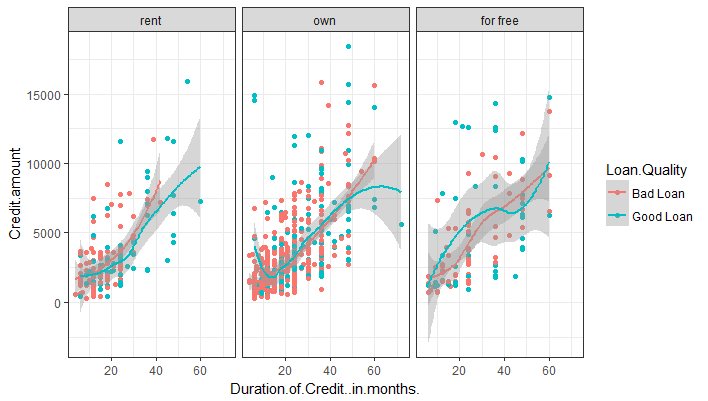### Modify/Override specific elements of the chosen theme

We can use the default theme or another built-in theme and then override some elements of it using the theme() function.

The visual elements can be classified as one of the three different types:

• Text
• Line
• Rectangle

Each type can be modified by calling the appropriate function, namely, element_text()element_line() and element_rect().

Let's take a few examples to understand how this works.

#### Change Plot Background to Light Yellow

By default, the entire plot has a white background. We will change to to light green color. The background color of the plot is defined by plot.background() in the default function. The background is a part of the rectangle shape, so we can modify it using the element_rect() function. We will change the background color to light yellow and add a gray border of size 2.

g <- ggplot(df,aes(x=Duration.of.Credit..in.months.,y=Credit.amount,color=Loan.Quality))
plot<-g+geom_point()+geom_smooth()+facet_wrap(~Type.of.housing)
plot+theme(plot.background = element_rect(fill = "#FFF6B0",color = "#737373", size = 2))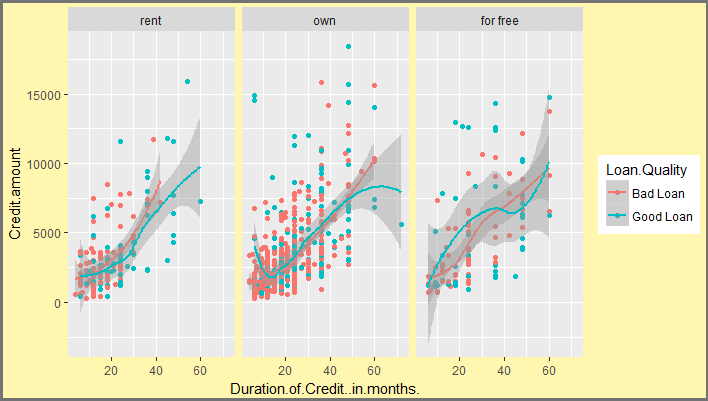#### Change Axis Line and Tick Color

The plot lines can be modified using the element_line() function. Take a look at the default theme function above. The axis lines are axis.line and axis ticks are defined with axis.ticks. Also notice that the panels have a grid which is defined by panel.grid. We will remove the grids by using element_blank().

plot+theme(plot.background = element_rect(fill = "#FFF6B0",color = "#737373", size = 2),
panel.grid = element_blank(),
axis.line = element_line(color = "#FF7E42"),
axis.ticks = element_line(color = "#FF7E42")
)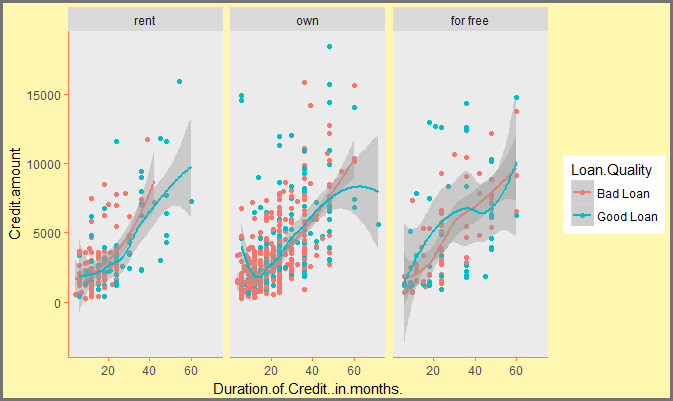#### Remove Panel and Legend Backgrounds

We will now go a step further and remove the backgrounds of the panels and the legends as shown below:

g <- ggplot(df,aes(x=Duration.of.Credit..in.months.,y=Credit.amount,color=Loan.Quality))
plot<-g+geom_point()+geom_smooth()+facet_wrap(~Type.of.housing)
plot+theme(plot.background = element_rect(fill = "#FFF6B0",color = "#737373", size = 2),
panel.grid = element_blank(),
axis.line = element_line(color = "#FF7E42"),
axis.ticks = element_line(color = "#FF7E42"),
panel.background = element_blank(),
legend.key = element_blank(),
legend.background=element_blank(),
strip.background = element_blank()
)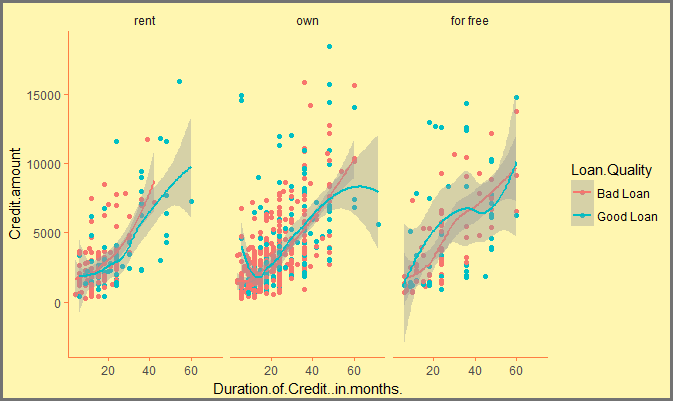#### Change Text in the Chart

We can make changes to the various text elements using the element_text() function. Let's make the following changes:

• Change strip text (text that appears in panels) to pink (#E1315B) and increase their size to 16.
• Change color of x-axis and y-axis titles to blue (#008DCB) and make them italics
plot+theme(plot.background = element_rect(fill = "#FFF6B0",color = "#737373", size = 2),
panel.grid = element_blank(),
axis.line = element_line(color = "#FF7E42"),
axis.ticks = element_line(color = "#FF7E42"),
panel.background = element_blank(),
legend.key = element_blank(),
legend.background=element_blank(),
strip.background = element_blank(),
strip.text = element_text(size = 16, color = "#E1315B"),
axis.title.y = element_text(color = "#008DCB", hjust = 0, face = "italic"),
axis.title.x = element_text(color = "#008DCB", hjust = 0, face = "italic"),
axis.text = element_text(color = "black")
)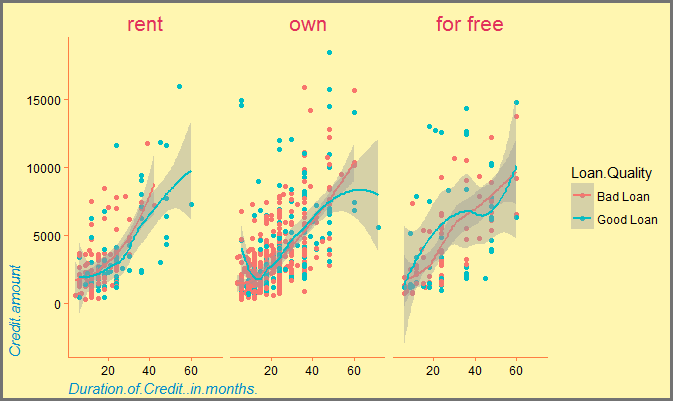#### Change Legend Position

We can modify various aspects of the legend using the theme() function. In the default theme, the legend has the following properties:

legend.background = theme_rect(colour="white"),
legend.key =        theme_rect(fill = "grey95", colour = "white"),
legend.key.size =   unit(1.2, "lines"),
legend.text =       theme_text(size = base_size * 0.8),
legend.title =      theme_text(size = base_size * 0.8, face = "bold", hjust = 0),
legend.position =   "right"


As you can see, the default position is right. The other options are 'bottom', 'left', 'top' or 'none'.

In the following example, we change the legend position to bottom.

plot+theme(plot.background = element_rect(fill = "#FFF6B0",color = "#737373", size = 2),
panel.grid = element_blank(),
axis.line = element_line(color = "#FF7E42"),
axis.ticks = element_line(color = "#FF7E42"),
panel.background = element_blank(),
legend.key = element_blank(),
legend.background=element_blank(),
strip.background = element_blank(),
strip.text = element_text(size = 16, color = "#E1315B"),
axis.title.y = element_text(color = "#008DCB", hjust = 0, face = "italic"),
axis.title.x = element_text(color = "#008DCB", hjust = 0, face = "italic"),
axis.text = element_text(color = "black"),
legend.position = "bottom"
)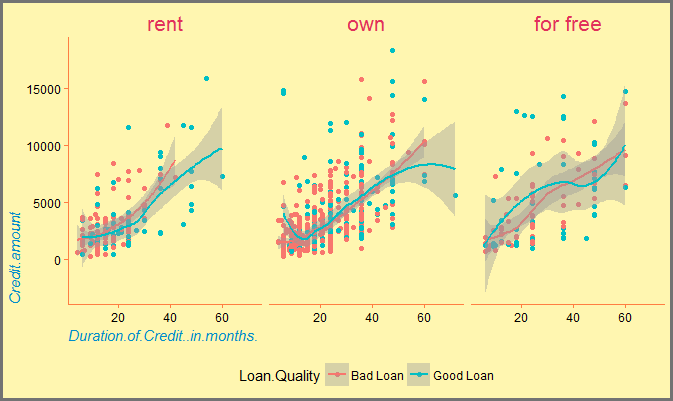This way we can modify almost every element of the ggplot and achieve any kind of styling we want.